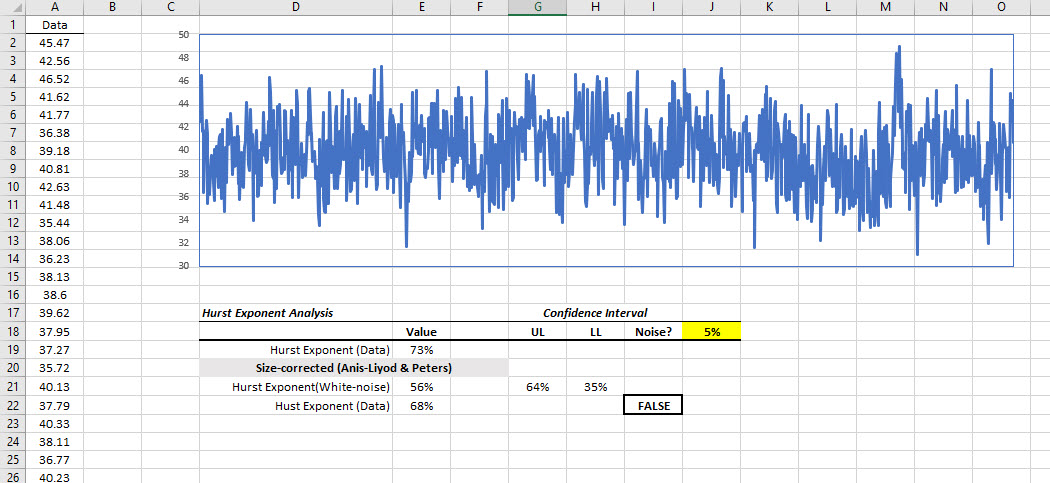# Hurst - Hurst Exponent, Index, or Parameter

Calculates the Hurst exponent (a measure of persistence or long memory) for time series with more than 96 observations.

## Syntax

Hurst(X, Alpha, Return_type)

X
is the input data sample (a one-dimensional array of cells (e.g., rows or columns)).
Alpha
is the statistical significance of the test (i.e., $\alpha$). If missing or omitted, an alpha value of 5% is assumed.
Return_type
is a number that determines the type of return value: 1 (or missing) = Empirical, 2 = (Anis-Lloyd/Peters) Corrected, 3 = Theoretical, 4 = Upper Limit, 5 = Lower Limit.
RETURN_TYPE NUMBER RETURNED
1 or omitted Empirical Hurst exponent.
2 Corrected Hurst exponent (Anis-Lloyd/Peters).
3 Theoretical Hurst exponent (Anis-Lloyd/Peters).
4 Upper limit of empirical confidence interval.
5 Lower limit of empirical confidence interval.

## Remarks

1. The input data series must have at least 96 non-missing values. Otherwise, the Hurst function returns #VALUE.
2. The input data series may include missing values (e.g., #N/A, #VALUE!, #NUM!, empty cell), but they will not be included in the calculations.
3. The Hurst exponent, $h$, is defined in terms of the Rescaled Range as follows: $$E \left [ \frac{R(n)}{S(n)} \right ]=Cn^H \qquad \mathrm{as} \ n \to \infty$$ Where:
• $\left [ \frac{R(n)}{S(n)} \right ]$ is the Rescaled Range.
• $E \left [x \right ]$ is the expected value.
• $n$ is the time of the last observation (e.g., it corresponds to $X_n$ in the input time series data).
• $h$ is a constant.
4. The Hurst exponent is a measure of autocorrelation (persistence and long memory).
• A value of $0 \lt H \lt 0.5$ indicates a time series with negative autocorrelation (e.g., a decrease between values will probably be followed by another decrease),
• A value of $0.5 \lt H \lt 1$ indicates a time series with positive autocorrelation (e.g., an increase between values will probably be followed by another increase),
• A value of $H=0.5$ indicates a "true random walk," where it is equally likely that a decrease or increase will follow from any particular value (e.g., the time series has no memory of previous values).
5. The Hurst exponent's namesake, Harold Edwin Hurst (1880-1978), was a British hydrologist who researched reservoir capacity along the Nile river.
6. The Rescaled Range is calculated for a time series, $X=X_1,X_2,\dots, X_n$, as follows:
1. Calculate the mean: $$m=\dfrac{1}{n} \sum_{i=1}^{n} X_i$$
2. Create a mean adjusted series: $$Y_t=X_{t}-m \qquad \mathrm{for}\ t=1,2, \dots ,n$$
3. Calculate the cumulative deviate series $Z$: $$Z_t= \sum_{i=1}^{t} Y_{i} \qquad \mathrm{for}\ t=1,2, \dots ,n$$
4. Create a range series $R$: $$R_t = max\left (Z_1, Z_2, \dots, Z_t \right )- min\left (Z_1, Z_2, \dots, Z_t \right ) \qquad \mathrm{for}\ t=1,2, \dots, n$$
5. Create a standard deviation series $R$: $$S_{t}= \sqrt{\dfrac{1}{t} \sum_{i=1}^{t}\left ( X_{i} - u \right )^{2}}\qquad \mathrm{for} \ t=1,2, \dots ,n$$ Where: $h$ is the mean for the time series values $X_1,X_2, \dots, X_t$.
6. Calculate the rescaled range series ($R/S$): $$\left ( R/S \right )_{t} = \frac{R_{t}}{S_{t}} \qquad \mathrm{for}\ t=1,2, \dots, n$$
7. The Hurst Exponent is estimated by fitting the power-law $E[R(n)/S(n)]=C\times n^H$ to the data. This is done by taking the logarithm of both sides and fitting a straight line. The slope of the line gives $H$ (i.e., Hurst Exponent Estimate).
8. The method above is known to produce a biased estimate of the power-law exponent, and for a small data set, there is a deviation from the 0.5 slope (i.e., white noise). Anis-LIoyd estimated the white-noise theoretical value of the $R/S$ statistics to be as follows: $$\operatorname {E} [R(n)/S(n)]={\begin{cases}{\frac {\Gamma ({\frac {n-1}{2}})}{{\sqrt {\pi }}\Gamma ({\frac {n}{2}})}}\sum \limits _{i=1}^{n-1}{\sqrt {\frac {n-i}{i}}},&{\text{for }}n\leq 340\\{\frac {1}{\sqrt {n{\frac {\pi }{2}}}}}\sum \limits _{i=1}^{n-1}{\sqrt {\frac {n-i}{i}}},&{\text{for }}n>340\end{cases}}$$ Where $\Gamma$ is the Euler Gamma Function.
9. No asymptotic distribution theory has been derived for most of the Hurst exponent estimators so far, but an approximate functional form for Anis-LIoyd corrected $R/S$ estimate is available. For a 95% confidence interval, the functional form for confidence interval limits is expressed as follows: $$LL=0.5 - \frac{e^{4.21}}{\ln(M)^{7.33}}$$ $$UL=0.5 + \frac{e^{4.77}}{\ln(M)^{3.10}}$$ Where $M = \log_2(N)$.
10. Finally, The Anis-Lloyd corrected $R/S$ Hurst exponent is calculated as 0.5 plus the slope of $R(n)/S(n)-\operatorname {E} [R(n)/S(n)]$.

## Illustration## References

•  A.A.Anis, E.H.Lloyd (1976) The expected value of the adjusted rescaled Hurst range of independent normal summands, Biometrica 63, 283-298.
•  H.E.Hurst (1951) Long-term storage capacity of reservoirs, Transactions of the American Society of Civil Engineers 116, 770-808.
•  E.E.Peters (1994) Fractal Market Analysis, Wiley.
•  R.Weron (2002) Estimating long-range dependence: finite sample properties and confidence intervals, Physica A 312, 285-299.
• Hamilton, J .D.; Time Series Analysis, Princeton University Press (1994), ISBN 0-691-04289-6.
• Tsay, Ruey S.; Analysis of Financial Time Series John Wiley & SONS. (2005), ISBN 0-471-690740.